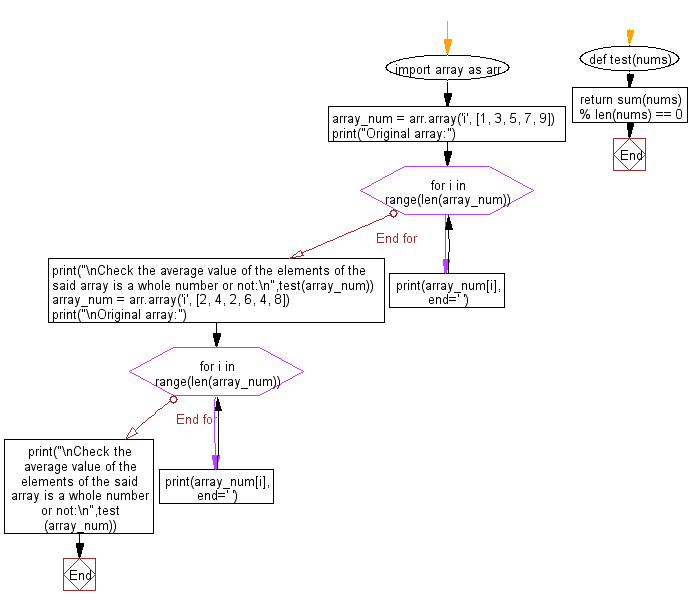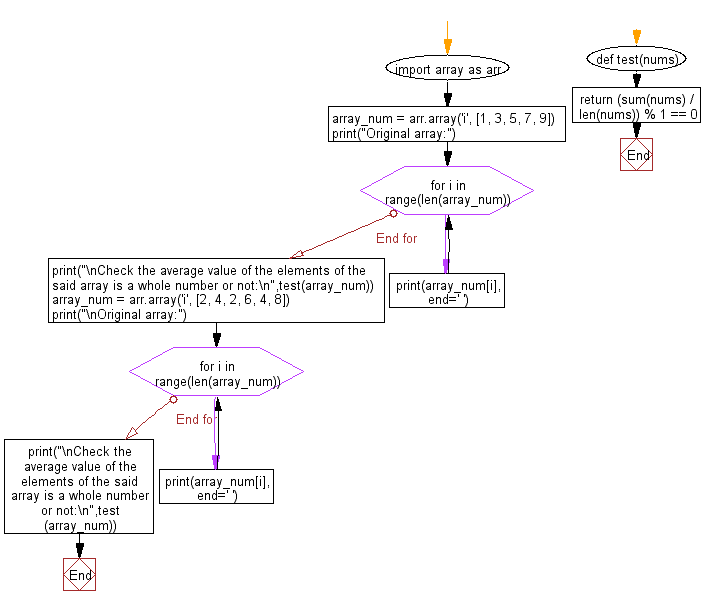﻿ Python: Check the average value of the elements of a given array of numbers is a whole number or not - w3resource# Python: Check the average value of the elements of a given array of numbers is a whole number or not

## Python Basic - 1: Exercise-127 with Solution

Write a Python program to check whether the average value of the elements of a given array of numbers is a whole number or not.

Sample Solution-1:

Python Code:

``````import array as arr
def test(nums):
return sum(nums) % len(nums) == 0
array_num = arr.array('i', [1, 3, 5, 7, 9])
print("Original array:")
for i in range(len(array_num)):
print(array_num[i], end=' ')
print("\nCheck the average value of the elements of the said array is a whole number or not:\n",test(array_num))
array_num = arr.array('i', [2, 4, 2, 6, 4, 8])
print("\nOriginal array:")
for i in range(len(array_num)):
print(array_num[i], end=' ')
print("\nCheck the average value of the elements of the said array is a whole number or not:\n",test(array_num))
``````

Sample Output:

```Original array:
1 3 5 7 9
Check the average value of the elements of the said array is a whole number or not:
True

Original array:
2 4 2 6 4 8
Check the average value of the elements of the said array is a whole number or not:
False
```

Flowchart:Sample Solution-2:

Python Code:

``````import array as arr
def test(nums):
return (sum(nums) / len(nums)) % 1 == 0
array_num = arr.array('i', [1, 3, 5, 7, 9])
print("Original array:")
for i in range(len(array_num)):
print(array_num[i], end=' ')
print("\nCheck the average value of the elements of the said array is a whole number or not:\n",test(array_num))
array_num = arr.array('i', [2, 4, 2, 6, 4, 8])
print("\nOriginal array:")
for i in range(len(array_num)):
print(array_num[i], end=' ')
print("\nCheck the average value of the elements of the said array is a whole number or not:\n",test(array_num))
``````

Sample Output:

```Original array:
1 3 5 7 9
Check the average value of the elements of the said array is a whole number or not:
True

Original array:
2 4 2 6 4 8
Check the average value of the elements of the said array is a whole number or not:
False
```

Flowchart:Python Code Editor:

Have another way to solve this solution? Contribute your code (and comments) through Disqus.

What is the difficulty level of this exercise?

Test your Programming skills with w3resource's quiz.

﻿

## Python: Tips of the Day

Iterating over dictionaries using 'for' loops:

I am a bit puzzled by the following code: d = {'x': 1, 'y': 2, 'z': 3} for key in d: print key, 'corresponds to', d[key] What I don't understand is the key portion. How does Python recognize ...

key is just a variable name.

```for key in d:
```

For Python 3.x:

```for key, value in d.items():
```

For Python 2.x:

```for key, value in d.iteritems():
```

To test for yourself, change the word key to poop.

In Python 3.x, iteritems() was replaced with simply items(), which returns a set-like view backed by the dict, like iteritems() but even better. This is also available in 2.7 as viewitems().

The operation items() will work for both 2 and 3, but in 2 it will return a list of the dictionary's (key, value) pairs, which will not reflect changes to the dict that happen after the items() call. If you want the 2.x behavior in 3.x, you can call list(d.items()).

Ref: https://bit.ly/37dm0Qo